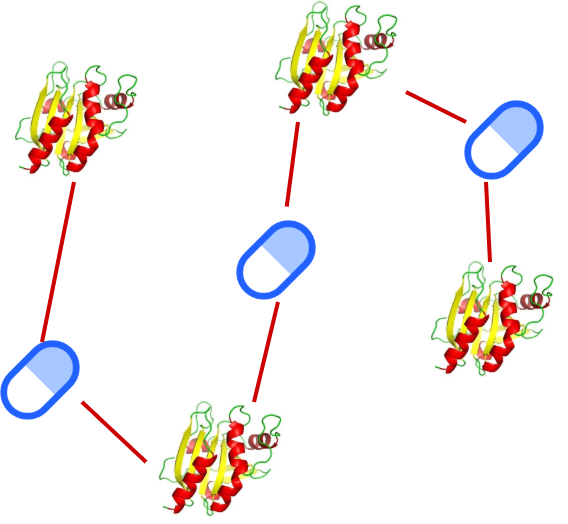# An Intuitive Explanation of NeoDTI

Source: Deep Learning on Medium# Introduction

In the previous stories of this series, we have discussed DeepWalk and GraphSAGE. Both of them were proposed to learn node representations in networks with a single entity and a single link type, i.e. homogenous networks. If you are unaware of these methods, you can check the previous stories.

In this story, we will explain NeoDTI , which has a different perspective. NeoDTI diverges from DeepWalk and GraphSAGE in two aspects. Firstly, NeoDTI is capable of operating on heterogeneous networks, i.e. networks with multiple link and entity types. Secondly, unlike DeepWalk and GraphSAGE, NeoDTI learns task-specific node embeddings, rather than general-purpose ones. In this manner, NeoDTI embeddings are specialized for a task, namely link prediction.

NeoDTI is proposed to utilize heterogeneous data for the drug-target interaction (DTI) prediction task. In the DTI task, each drug and target is represented with a node and two nodes are adjacent if they are known to be interacting. Therefore, link prediction corresponds to predict whether a drug and a protein interact. Below we can see a toy heterogeneous network that consists of three drugs and four targets.

Although NeoDTI is proposed for DTI, link prediction on heterogeneous networks is a cross-domain problem. For instance, consider the Medium network where users and stories are represented as nodes. In this network, an edge between users denotes follower relation, whereas an edge between user and story denotes clapping. In this scenario, the link prediction task can be used for either friend or story recommendation to users.

The Medium network example is prominent to emphasize the generalizability of NeoDTI. Yet, we will stick to the DTI prediction task for the rest of the story to comply with the original paper. We will describe NeoDTI in three steps as neighborhood integration, node embedding update, and edge reconstruction learning.

# Neighborhood Integration

NeoDTI works on a network with four entities and twelve relations. The entities are drugs, targets, diseases, and side effects whereas the relations contain the interaction, association, and similarities of these entities. Each relation is represented with binary edges. Neighborhood integration aims to combine multi-aspect information in the neighborhood of a node and reflect it in the node’s embedding.

Despite the idea is quite similar to the ones in DeepWalk and GraphSAGE, NeoDTI follows a different strategy. Unlike previous methods, NeoDTI does not explore the node’s neighborhood by graph traversals, but it considers all adjacent nodes as the neighborhood. For each entity type in the neighborhood, NeoDTI passes each adjacent node through a nonlinear neural network layer and apply a normalizer to their sum to obtain an entity type specific neighborhood embedding. To combine information from different entity types, NeoDTI applies summation and obtains the resulting neighborhood embedding. Below we can see the neighborhood integration equation for a node v.

In this equation, u is an adjacent node to v with an edge type r and f ⁰(u) is a function that retrieves the initial embedding vector of u. Wᵣ and bᵣ are edge type specific neural network weights and σ is the nonlinear activation function. The coefficient in front of the neural network is a normalizer based on node degree. Lastly, aᵥ is the resulting neighborhood embedding.

# Node Embedding Update

Having constructed the neighborhood embedding for a node v, it is time to combine it with the v’s own embedding. To do so, NeoDTI concatenates the initial embedding of v (f ⁰ (v)) with the neighborhood embedding (aᵥ). Similar to the previous step, the concatenated vector is passed through another nonlinear neural network layer and then normalized to be unit length to obtain the updated node embedding (f ¹(v)). In the equation below, and denote the weights of the neural network at this step.

In theory, the update step can be repeated several times to create more embeddings. However, in practice using a single update step is sufficient to obtain reasonably good predictions.

# Edge Reconstruction Learning

In the previous steps, we defined the necessary procedures to create node embeddings with heterogeneous neighborhood information. Now it is time to define a loss function to realize the learning. NeoDTI uses a loss function to promote edge reconstruction. It other words, NeoDTI aims to reconstruct edges of the initial network from the node embeddings.

Below we see the loss formulation, where Gᵣ and Hᵣ are edge type specific projection matrices and s(e) weight of the edge between u and v. To create negative samples, NeoDTI samples nonadjacent nodes and provides them as input as well during the training.

Now let us interpret the loss function. To minimize the loss, the term inside the summation should be as close as possible to 0. Thus, s(e) must be equal to f ¹(u)ᵀ Gᵣ Hᵣᵀ f ¹(v). Since f ¹(u)ᵀ Gᵣ Hᵣᵀ f ¹(v) is a reconstruction term based on node embeddings and projection matrices, embeddings are learned such that edges are reconstructed.

As opposed to DeepWalk and GraphSAGE which aims to maximize embedding similarity between similar nodes, NeoDTI embeddings are learned for edge reconstruction. This is what makes them task-specific.

With this formulation, the reconstruction term can be used to sample predictions of NeoDTI. To predict whether a drug u and target v interacts, we compute the reconstruction term using learned drug and target projection matrices, f ¹(u), and f ¹(v). The result is the prediction of NeoDTI for the edge weight between u and v.

# Discussion

NeoDTI is an end-to-end learning method to learn task-specific node embeddings. Both embedding creation and loss function steps are fully differentiable and all weights and embeddings can be learned via gradient descent. The resulting embeddings of this end-to-end procedure are optimized for edge reconstruction i.e. link prediction. Hence, the generalizability of the learned embeddings is sacrificed, in the face of an increase in link prediction accuracy. Below we can see the workflow of NeoDTI in a single figure.